# Circuit adds programmability to sensor amplifier

## ON Semiconductor CAT5113 CAT5114

The pressure-sensor amplifier circuit of Figure 1 offers a number of advantages over the traditional approach using the classic three-op-amp instrumentation amplifier. The circuit can operate from a single supply and uses only two op amps and 1% resistors. If the reference voltage, VREF, is 0 V, the transducer gain for the circuit is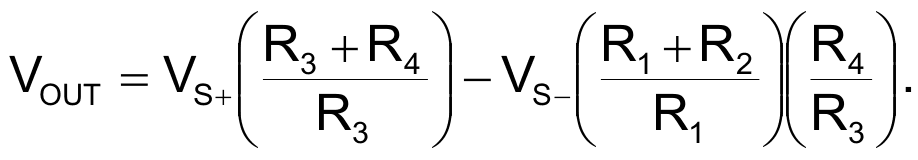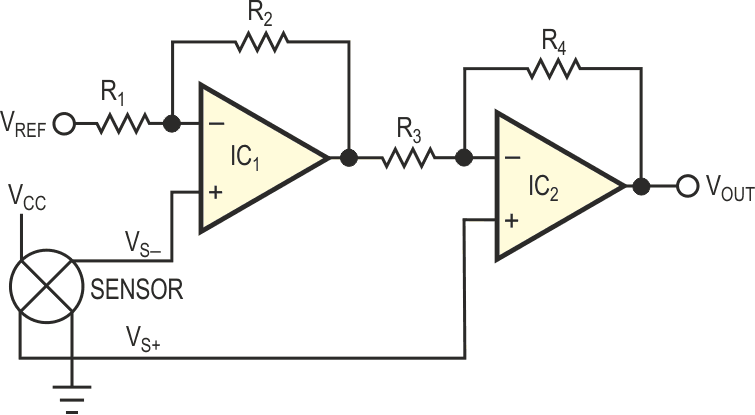Figure 1. This circuit is a good sensor amplifier but suffers from a lack of variability.

To ensure equal gain for the two ground-referenced voltages making up the differential sensor voltage, you must impose the following condition: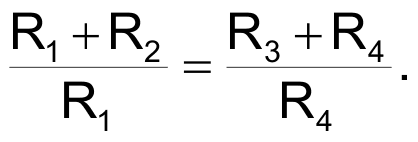This restriction complicates the design and makes it difficult to add variability to the circuit. The circuit in Figure 2 has the same advantageous topology as the original circuit, but it adds variability and programmability to the gain, simplifies the design expressions, and reduces the component count. Two ganged digitally programmable potentiometers replace resistors R1 through R4. The potentiometers' wiper-to-low and wiper-to-high resistances establish the voltage gains of IC1 and IC2. Because the potentiometers are identical, the end-to-end resistances are the same, thus meeting the (R1 + R2) = (R3 + R4) requirement. If you reverse the wiper-to-low and wiper-to-high resistances for IC1 and IC2, R1 and R4 are equal. If you mathematically model the wiper-to-low and wiper-to-high resistances as (1–p1)R and (p1)R, the gain expression becomes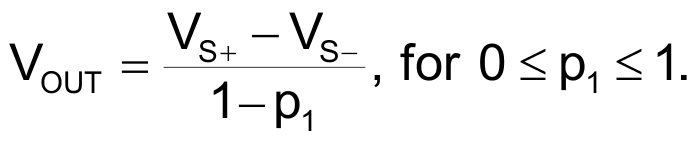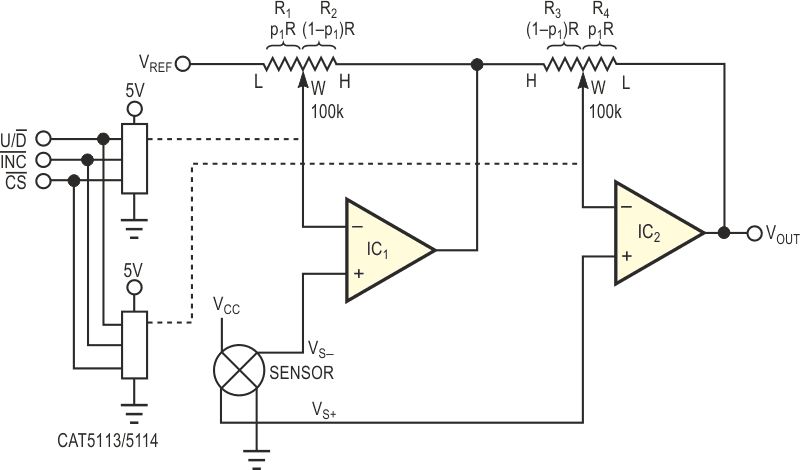Figure 2. An improved circuit features programmability and fewer components.

The factor p1 models the relative position of the wiper as it moves from one end (p1 = 0) of the potentiometer to the other end (p1 = 1). The number of values p1 can assume is a function of the number of taps on the potentiometer. The sensor gain is inversely proportional to (1–p1), is pseudo-logarithmic, and varies from less than unity to 31 for a 32-tap potentiometer, such as the CAT5114, and to 99 for a 100-tap potentiometer, such as the CAT5113. The voltage, VREF, represents a programmable offset voltage for the signal-conditioning circuit. You can easily implement the reference voltage by using a digitally programmable potentiometer configured as a programmable voltage divider.

EDN